# Samacheer Kalvi 8th Maths Solutions Term 2 Chapter 3 Geometry Ex 3.4

Students can Download Maths Chapter 3 Geometry Ex 3.4 Questions and Answers, Notes Pdf, Samacheer Kalvi 8th Maths Book Solutions Guide Pdf helps you to revise the complete Tamilnadu State Board New Syllabus and score more marks in your examinations.

## Tamilnadu Samacheer Kalvi 8th Maths Solutions Term 2 Chapter 3 Geometry Ex 3.4

Question I.
Construct the following parallelograms with the given measurements and find their area.

Question 1.
ARTS, AR = 6 cm, RT = 5 cm and ∠ART = 70°.
Solution: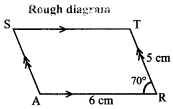Given:
In the Parallelogram ARTS,
AR = 6 cm
RT = 5 cm and ∠ART = 70°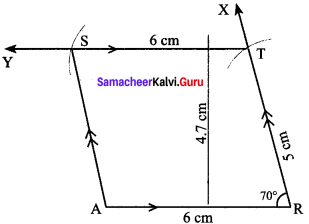Construction:
Steps:

1. Draw a line segment AR = 6 cm.
2. Make an angle ∠ART = 70° at R on AR
3. With R as centre, draw an arc of radius 5 cm cutting RX at T
4. Draw a line TY parallel to AR through T.
5. With T as centre, draw an arc of radius 6 cm cutting TY at S. Join AS
6. ARTS is the required parallelogram.

Calculation of area:
Area of the parallelogram ARTS = b x h sq. units
= 6 x 4.7 = 28.2 sq. cmQuestion 2.
BANK, BA = 7 cm, BK = 5.6 cm and ∠KBA = 85°.
Solution: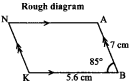Given:
In the parallelogram BANK, BA =7 cm, BK = 5.6 cm, and ∠KBA = 85°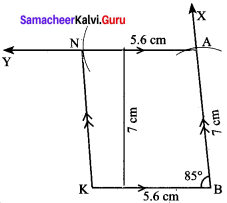Construction:
Steps:

1. Draw a line segment KB = 5.6 cm.
2. Make an angle ∠KBA = 85° at B
3. Draw an arc of radius 7 cm with B as centre on BX.
4. Draw a line AY parallel to KB.
5. With A as centre, draw an arc of radius 5.6 cm cutting AY at N. Join KN
6. BANK is the required parallelogram.

Calculation of area:
Area of the Parallelogram BANK = b x h sq. units
= 5.6 x 7 = 39.2 sq. cm

Question 3.
CAMP, CA = 6 cm, AP = 8 cm and CP = 5.5 cm.
Solution: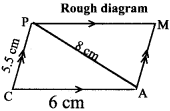Given:
In the parallelogram CAMP,
CA = 6 cm
AP = 8 cm, and CP = 5.5cm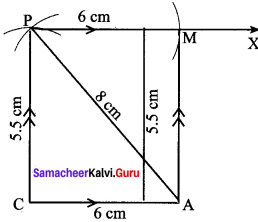Construction:
Steps:

1. Draw a line segment CA = 6 cm.
2. With C as centre, draw an arc of length 5.5 cm
3. With A as centre, draw an arc of length 8 cm
4. Mark the intersecting point of these two arcs as P
5. Draw a line PX parallel to CA
6. With P as centre draw an arc of radius 6 cm cutting PX at M. Join AM
7. CAMP is the required parallelogram.

Calculation of area:
Area of the Parallelogram CAMP = b x h sq. units
= 6 x 5.5 = 33 sq. cm

Question 4.
DRUM, DR = 7 cm, RU = 5.5 cm and DU = 8 cm.
Solution: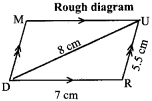Given:
In the parallelogram DRUM,
DR = 7 cm,
RU = 5.5 cm, and DU = 8 cm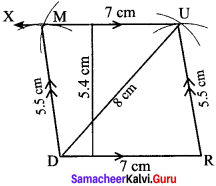Construction:
Steps:

1. Draw a line segment DR = 7 cm.
2. With D and R as centres, draw arcs of radii 8 cm and 5.5 cm.
3. Mark the intersecting point of these arcs as U. Join DU and RU
4. Draw a line UX parallel to DR through U.
5. With U as centre draw an arc of radius 7 cm cutting UX at M. Join DM
6. DRUM is the required parallelogram.

Calculation of area:
Area of the Parallelogram DRUM = b x h sq. units
= 7 x 5.4 = 37.8 sq. cmQuestion 5.
EARN, ER = 10 cm, AN = 7 cm and ∠EOA 110° where $$\overline { ER }$$ and $$\overline { AN }$$ intersect at O.
Solution: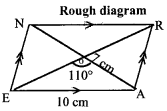Given:
In the parallelogram EARN,
ER= 10 cm
AN = 7 cm, and ∠EOA = 110°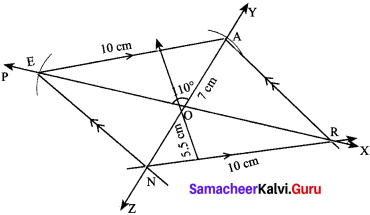Construction:
Steps:

1. Draw a line segment PX. Mark a {Joint O on PX
2. Make an angle ∠EOA = 110° on PX at O
3. Draw arcs of radius 3.5 cm with O as centre on either side of PX. Cutting YZ on A and N
4. With A as centre, draw an arc of radius 10 cm, cutting PX at E. Join AE
5. Draw a line parallel to AE at N cutting PX at R. Join EN and AR
6. EARN is the required parallelogram

Calculation of area:
Area of the Parallelogram EARN = b x h sq. units
= 10 x 5.5 = 55 sq. cm

Question 6.
FAIR, FI = 8 cm, AR = 6 cm and ∠IOR = 80° where $$\overline { FI }$$ and $$\overline { AR }$$ intersect at O.
Solution: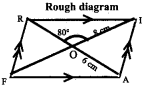Given:
In the parallelogram FAIR,
FI = 8cm
AR = 6 cm and ∠IOR = 80°, where $$\overline { FI }$$ and $$\overline { AR }$$ intersect at O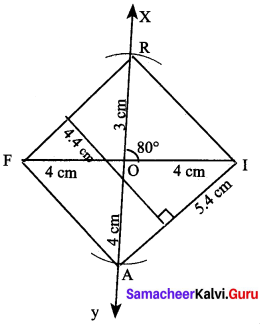Construction:
Steps:

1. Draw a line segment $$\overline { FI }$$ = 8 cm
2. Mark O the midpoint of $$\overline { FI }$$
3. Draw a line $$\overline { XY }$$ through O which makes ∠IOX = 80°
4. With O as centre and 3 cm as radius draw two arcs on XY on either sides of $$\overline { FI }$$. Let the arcs cut $$\overline { OX }$$ at R and $$\overline { OY }$$ at A
5. Join $$\overline { IR }$$, $$\overline { FR }$$, $$\overline { FA }$$ and $$\overline { AI }$$
6. FAIR is the required parallelogram

Calculation of area:
Area of the Parallelogram FAIR = b x h sq. units
= 5.4 x 4.3 = 23.22 sq. cm

Question 7.
GAIN, GA = 7.5 cm, GI = 9 cm and ∠GAI – 100°
Solution: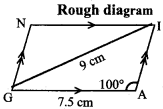Given:
In the parallelogram GAIN,
GA = 7.5 cm
GI = 9 cm and ∠GAI= 100°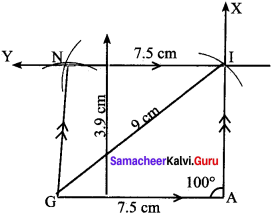Construction:
Steps:

1. Draw a line segment GA = 7.5 cm.
2. Make an angle GAI = 100° at A.
3. With G as centre, draw an arc of radius 9 cm cutting AX at I. Join GI.
4. Draw a line IY parallel to GA through I.
5. With I as centre, draw an arc of radius T.5 cm on IY cutting at N. Join GN
6. GAIN is the required parallelogram.

Calculation of area:
Area of Parallelogram GAIN = b x h sq.units
= 7.5 x 3.9 = 29.25 sq. cmQuestion 8.
HERB, HE = 6 cm, ∠EHB = 60° and EB = 7 cm.
Solution: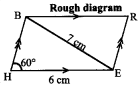Given:
In the parallelogram HERB,
HE = 6 cm, ∠EHB = 60° and
EB = 7 cm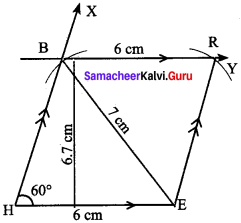Construction:
Steps:

1. Draw a line segment HE = 6 cm.
2. Make an angle ∠BHE = 60° at H.
3. With E as centre, draw an arc of radius 7 cm cutting HX at B.
4. Draw a line BY parallel to HE through B.
5. Draw an arc of radius 6 cm with B as centre, cutting BY at R. Join ER.
6. HERB is the required parallelogram.

Calculation of area:
Area of the Parallelogram HERB = b x h sq. units
= 6 x 6.7 = 40.2 sq. cm